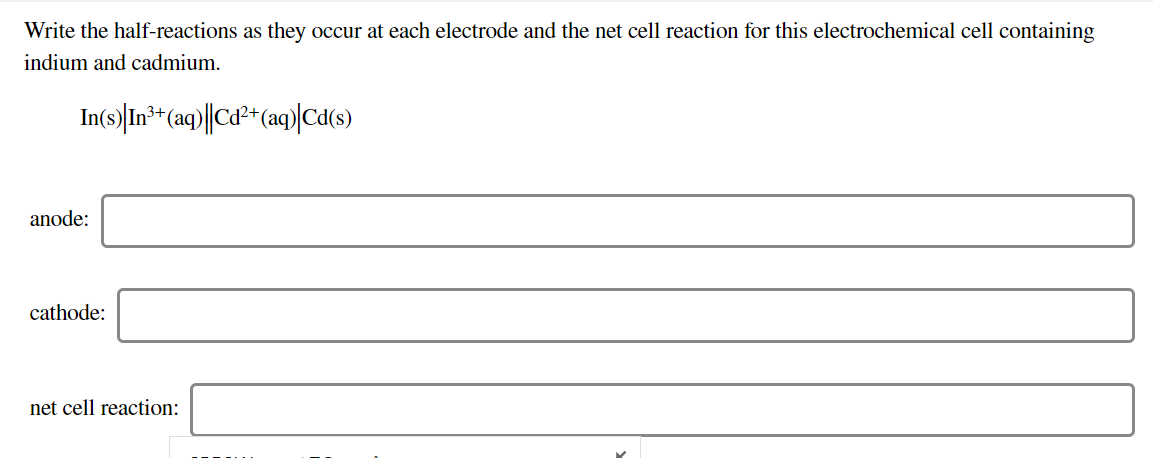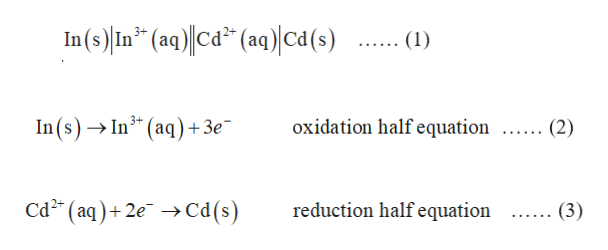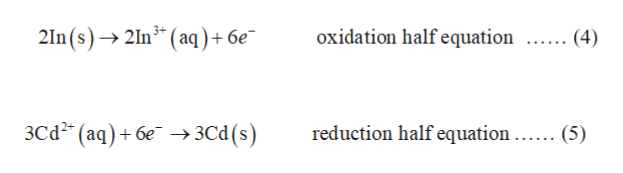# Write the half-reactions as they occur at each electrode and the net cell reaction for this electrochemical cell containingindium and cadmiumIn(s) In* (aq)|Ca2 (ag)Ca(s)3+anodecathodenet cell reaction:

Question
45 viewshelp_outlineImage TranscriptioncloseWrite the half-reactions as they occur at each electrode and the net cell reaction for this electrochemical cell containing indium and cadmium In(s) In* (aq)|Ca2 (ag)Ca(s) 3+ anode cathode net cell reaction: fullscreen
check_circle

Step 1

The given cell reaction by shorthand notation is labelled as equation (1).

The half-cell reaction at anode is given as equation (2).

The half-cell reaction at cathode is given as equation (3).help_outlineImage Transcriptionclose... (1 In(s) In* (aq)Ca2 (aq)ca(s) .... (2) oxidation half equation In (s)In3(aq)+3e (3) reduction half equation Cd2 (aq)2e>Cd (s) fullscreen
Step 2

Multiply equation (2) by  2 as shown in equation (4).

Multi...help_outlineImage Transcriptionclose2In(s)2In3 (aq) + 6e"| oxidation half equation (4) 3Cd2 (aq) 6e->3Cd(s reduction half equation ...... (5) fullscreen

### Want to see the full answer?

See Solution

#### Want to see this answer and more?

Solutions are written by subject experts who are available 24/7. Questions are typically answered within 1 hour.*

See Solution
*Response times may vary by subject and question.
Tagged in

### Electrochemistry